This page has been robot translated, sorry for typos if any. Original content here.

# INFLUENCE OF ERROR OF TRANSFORMERS

The decline in production in recent years has led to a decrease in loads in a number of nodes of the power system, as well as a reduction in industrial consumption, which in turn has caused a negative error in automated energy control and metering systems (ASKUE). The reason for this was the occurrence of a negative error in the primary current and voltage sensors, which are used as current and voltage transformers. This work is devoted to the study of the causes of errors and ways to eliminate underestimation of power consumption in ASKUE systems.

Errors of current transformers (current and angular) are due to the presence of magnetization current and are calculated by the formulas [1, 2]:

current error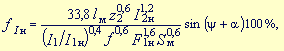(one)

where lm is the average length of the magnetic flux in the magnetic circuit, m; z2 — resistance of the secondary current branch (total resistance of the secondary circuit and secondary winding), Ohm; f is the frequency of the alternating current, Hz; Sm - the actual cross section of the magnetic circuit, m2; j is the angle of loss, and a is the phase angle between the secondary emf E2 and secondary current I2, deg .;

angular error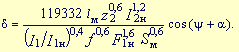(2)

The main influence on the error values ​​of current transformers is exerted by their current load and the resistance value of the secondary circuit. In the face of a decrease in electricity consumption by industrial enterprises, the load of current transformers often does not exceed 5 - 15%, which leads to a significant increase in errors.

The limiting values ​​of the current and angular errors of current transformers for measurements (according to GOST 7746-89) are given in the table.

 Accuracy class Primary current,% of rated Marginal error Secondary load,% of rated, at cos j 2= 0,8 current angular min hail 0.2 5 10 twenty 100-200 ± 0.75 ± 0.50 ± 0.35 ± 0.20 ± 30 ± 20 ± 15 ± 10 ± 0.9 ± 0.6 ± 0.4 ± 0.3 0.5 5 10 twenty 100-200 ± 1.5 ± 1.0 ± 0.75 ± 0.5 ± 90 ± 60 ± 45 ± 30 ± 2.5 ± 1.7 ± 1.35 ± 0.9 25-100

The results of calculating the angular and current errors of current transformers of the TPOL 600/5 type, accuracy class 0.5, produced by formulas (1) and (2), are shown in Fig. 1 and 2 (thin line - calculated curve, bold line - approximation). The type of the approximating expression and the criterion of agreement between the calculated and approximating curves are presented in the figures.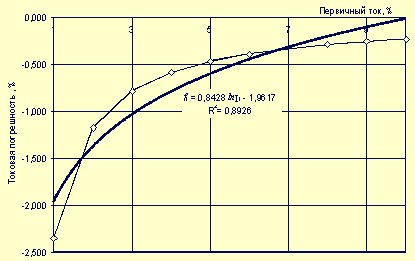Fig. one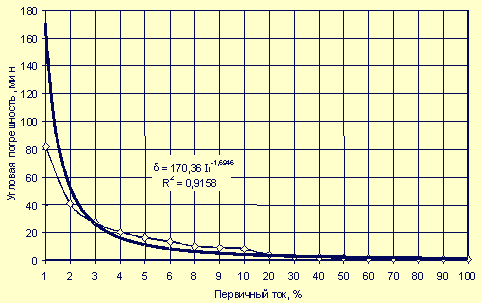Fig. 2

For ranges of change (1 - 10% and 10 - 100%) of the primary current from the nominal value, the mathematical models of the current error of the most common current transformers are:

TPOL10-600 / 5
Df [%] = 0.8428 * ln I1 - 1.9617 for 1 <I1 <10%
Df [%] = 0.0841 * ln I1 - 0.3919 for 10 <I1 <100%

TLSh10-2000 / 5
Df [%] = 0.7227 * ln I1 - 1.6815 for 1 <I1 <10%
Df [%] = 0.0722 * ln I1 - 0.3353 for 10 <I1 <100%

TPShFD10 - 3000/5
Df [%] = 0.5986 * ln I1 - 1.2261 for 1 <I1 <10%
Df [%] = 0.0597 * ln I1 - 0.1111 for 10 <I1 <100%

The values ​​of the primary current I1 of the current transformer in the formulas should be substituted as a percentage of the nominal value.

Studies of errors in current transformers conducted at the SRCE have shown acceptable convergence of theoretical and experimental results. In fig. 3 shows the results of an experimental study of TT type TPLM10-200 / 5, accuracy class 0.5.

The results of the study of current errors of various types of current transformers with a primary rated current of 75 - 600 A made it possible to draw the following conclusions: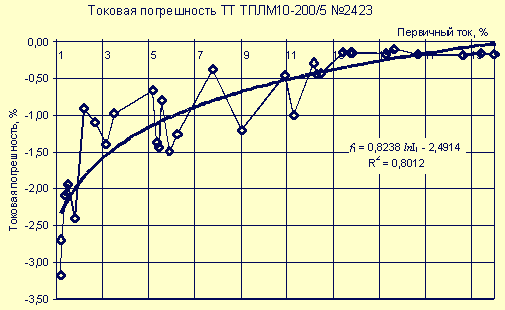Fig. 3

1. in the range of variation of the primary current from the nominal value of 1 - 25%, the current error has a negative sign;
2. with increasing primary current, the absolute value of the current error decreases;
3. experimental studies confirm the correctness of the mathematical model of the current error of the current transformer;
4. taking into account the current error of the current transformer in ASKUE will reduce the amount of unbalance in substations;
5. the amount of electricity supplied to consumers due to the negative current error of current transformers is underestimated in comparison with the actual value; therefore, taking into account the current error of the current transformer in ASKUE will allow more accurately assessing the amount of electricity supplied to consumers and obtain a certain economic effect, which will be evaluated further.

The second source of energy measurement error is a voltage transformer.

According to [3, 4], the voltage error is determined as follows: DU = DUн + DUх (3)
where DUн - voltage error due to load current,%; DUх - voltage error due to open circuit current,%.

Using a vector diagram, it is possible with sufficient accuracy to express the components of the error of the voltage transformer as follows: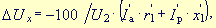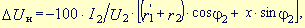where U2 is the voltage of the secondary winding of the transformer, V; Ia- active component of the open-circuit current, reduced to the secondary winding of the transformer, A; r'1 - reduced resistance of the primary winding of the transformer, reduced to the secondary winding, Ohm; I'p - reduced reactive component of the open-circuit current, reduced to the secondary winding of the transformer, A; x'1 - reactance of the primary winding of the transformer, reduced to the secondary winding, Ohm; I2 - load current of the transformer, A; r2 is the resistance of the secondary winding of the transformer, Ohm; cosj2 - load power factor, rel. units; x is the inductive resistance of the transformer, Ohm.

The angular error of the voltage transformer is defined as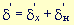,
where d'x is the angular error due to the open circuit current; d'n - angular error due to the load current.

The components of the angular error are defined as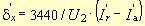;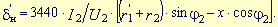The results of calculating the errors of the voltage transformer are shown in Fig. 4 and 5. The main influence on the error of the voltage transformer is exerted by the value of the secondary load I2.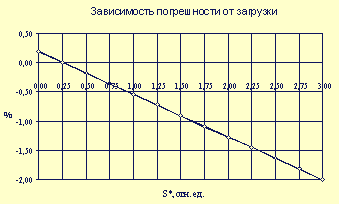Fig. 4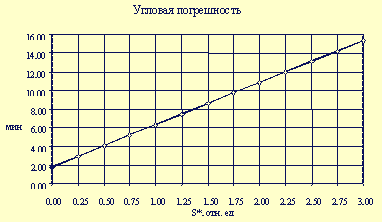Fig. 5

The dependence of the error of the voltage transformer on the load factor for power (the ratio of the actual load of the secondary winding of the voltage transformer to the nominal value of the load) has the form

DU [%] = - 0.73 * KZ + 0.35,
where KZ is the load of the voltage transformer in the secondary winding, rel. units

The obtained expressions for the errors of current transformers and voltage transformers can increase the accuracy of electricity metering at substations.

The effectiveness of the implementation of ASKUE in substations depends

• from the costs of introducing ASKUE;
• from the economic effect obtained as a result of implementation.

Currently, the metering of electricity supplied and the calculation of the energy balance at most substations is carried out using electromagnetic meters without taking into account the errors of current transformers and voltage transformers.

Often voltage transformers operate when loading the secondary winding, which exceeds the nominal one several times, i.e. with a negative error. Most of the load of the voltage transformer is made up of measuring devices connected to them, in particular electromagnetic active energy meters. For example, at the Sverdlovskaya substation, induction meters of the SAZU-I670M type are installed, the power consumption of which is 4 W. As a result of the introduction of ASKUE, induction meters will be replaced by electronic meters such as PSCh, the power consumption of which is two times less - 2 watts.

In this case, the load factor of the voltage transformer is halved to a value of 1.1 and, therefore, the error of the voltage transformer is reduced from 1.15% to 0.5%. Reducing the error of the voltage transformer will lead to an increase in the accuracy of accounting for the electricity supplied to consumers.

Taking into account current errors of current and voltage transformers in the ASKUE system gives an economic effect. To assess the economic effect of the introduction of ASCAE, an estimated calculation of the annual electricity consumption at the Sverdlovskaya substation was made taking into account the errors of current and voltage transformers. The calculation was carried out as follows:

1. According to available data for the characteristic winter and summer days of the year (1997 and 1998), hourly values ​​of active power were calculated (taking into account the errors of current and voltage transformers) from inputs and outgoing lines according to the formula

Rfact = P * KI * KU,
where P is the average hourly power values ​​determined by the readings of electric meters;
KI - coefficient taking into account the current error of the current transformer, KU - coefficient taking into account the error of the voltage transformer.

KI = 1 - (Df I/ 100), KU = 1 - (Df U/ 100),
where Df Iis the current error of the current transformer, Df Uis the error of the voltage transformer.

2. Electricity consumption was determined for typical winter and summer days, taking into account the errors of current and voltage transformers (Wz.act and Wl.fact) and without taking into account errors (Wz and Wl) for inputs and outgoing lines:

Wz.fact = S Rfact.z,
Wl.fact = S Rfact.l,
Wz = S Pz,
Wl = S Rl.

3. The value of the annual consumption of active electricity was calculated by inputs and outgoing lines according to the formulas

Wg fact = Wz fact * Nz + Wl fact * Nl
Wg = Wz * Nz + Wl * Nl,
where Nz = 213 and Nl = 152 - the number of winter and summer days in a year.

4. The effect of the introduction of ASKUE is determined by the formula DW = SWg.fact - SWg,
where SW.fact and SWg are the annual electricity consumption by the outgoing feeders, taking into account and without taking into account the errors of current and voltage transformers, respectively.

We will evaluate the economic effect for two options.

1. When taking into account AMC current errors of current transformers and reducing errors of voltage transformers due to the reduced power consumption of electronic meters, the effect will be:

according to 1997
DW = 331021094-326683013 = 4338081 kWh / year;

according to 1998
DW = 294647641-290512594 = 4135047 kWh / year.

In monetary terms, the economic effect (E) is equal (when the cost of electricity is 0.4 rubles / kWh)
E = 1735 ... 1650 thousand rubles per year.

2. Considering only the reduction of errors in voltage transformers due to the reduced power consumption of electronic meters, the effect will be:

according to 1997
DW = 328316428-326683013 = 1633415 kWh / year;

according to 1998
DW = 292196976-290512594 = 1684382 kWh / year.

In monetary terms, the economic effect is equal (when the cost of electricity is 0.4 rubles / kWh)
E = 653 ... 674 thousand rubles per year.

In conclusion, we can draw the following conclusions:

• reduction of loads in a number of nodes of the power system, as well as a decrease in electricity consumption by industry, led to the appearance of a negative error in current transformers and, accordingly, to the commercial underestimation of energy consumption;
• to eliminate underestimation of electricity consumption, it is necessary to introduce correction factors;
• taking into account errors of current transformers in ASKUE, as well as reducing voltage transformer errors due to the introduction of new electronic meters, lead to a significant economic effect.